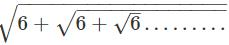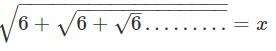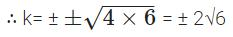Courses

## 20 Questions MCQ Test | Test: Quadratic Equations (Medium)

Description
This mock test of Test: Quadratic Equations (Medium) for Class 10 helps you for every Class 10 entrance exam. This contains 20 Multiple Choice Questions for Class 10 Test: Quadratic Equations (Medium) (mcq) to study with solutions a complete question bank. The solved questions answers in this Test: Quadratic Equations (Medium) quiz give you a good mix of easy questions and tough questions. Class 10 students definitely take this Test: Quadratic Equations (Medium) exercise for a better result in the exam. You can find other Test: Quadratic Equations (Medium) extra questions, long questions & short questions for Class 10 on EduRev as well by searching above.
QUESTION: 1

### The sum of two numbers is 27 and product is 182. The numbers are:

Solution: Let x is one number

Another number = 27 – x

Product of two numbers = 182 x(27 – x) = 182

⇒ x2 – 27x – 182 = 0

⇒ x2 – 13x – 14x + 182 = 0

⇒ x(x – 13) -14(x – 13) = 0

⇒ (x – 13)(x -14) = 0

⇒ x = 13 or x = 14

QUESTION: 2

### If 1 / 2 is a root of the quadratic equation x2 - mx - 5 / 4 = 0, then value of m is:

Solution: Given x = 1 / 2 as root of equation x2 - mx - 5 / 4 = 0.

(1 / 2)2 – m(1 / 2) – 5 / 4 = 0

1 / 4 - m / 2 - 5 / 4 = 0

m = -2

QUESTION: 3

### The altitude of a right triangle is 7 cm less than its base. If the hypotenuse is 13 cm, the other two sides of the triangle are equal to:

Solution: Let the base be x cm.

Altitude = (x – 7) cm

In a right triangle,

Base2 + Altitude2 = Hypotenuse2 (From Pythagoras theorem)

∴ x2 + (x – 7)2 = 132

By solving the above equation, we get;

⇒ x = 12 or x = – 5

Since the side of the triangle cannot be negative.

Therefore, base = 12cm and altitude = 12 - 7 = 5cm

QUESTION: 4

The roots of quadratic equation 2x2 + x + 4 = 0 are:

Solution: 2x2 + x + 4 = 0

⇒ 2x2 + x = -4

Dividing the equation by 2, we get

⇒ x2 + 1 / 2x = -2

⇒ x2 + 2 × x × 1 / 4 = -2

By adding (1 / 4)2 to both sides of the equation, we get

⇒ (x)2 + 2 × x × 1 / 4 + (1 / 4)2 = (1 / 4)2 – 2

⇒ (x + 1 / 4)2 = 1 / 16 – 2

⇒ (x + 1 / 4)2 = -31 / 16

The square root of negative number is imaginary, therefore, there is no real root for the given equation.

QUESTION: 5

The value ofis:

Solution: Let,Hence, we can write, √(6 + x) = x

6 + x = x2

x2 - x - 6 = 0

x2 - 3x + 2x - 6 = 0

x(x - 3) + 2(x - 3) = 0

(x + 2) (x - 3) = 0

x = -2, 3

Since, x cannot be negative, therefore, x = 3

QUESTION: 6

The sum of the reciprocals of Rehman’s ages 3 years ago and 5 years from now is 1 / 3. The present age of Rehman is:

Solution: Let, x is the present age of Rehman

Three years ago his age = x – 3

Five years later his age = x + 5

Given, the sum of the reciprocals of Rehman’s ages 3 years ago and after 5 years is equal to 1 / 3.

∴ 1 / x - 3 + 1 / x - 5 = 1 / 3

(x + 5 + x - 3) / (x - 3)(x + 5) = 1 / 3

(2x + 2) / (x - 3)(x + 5) = 1 / 3

⇒ 3(2x + 2) = (x - 3)(x + 5)

⇒ 6x + 6 = x2 + 2x – 15

⇒ x2 – 4x – 21 = 0

⇒ x2 – 7x + 3x – 21 = 0

⇒ x(x – 7) + 3(x – 7) = 0

⇒ (x – 7)(x + 3) = 0

⇒ x = 7, -3

We know age cannot be negative, hence the answer is 7.

QUESTION: 7

A train travels 360 km at a uniform speed. If the speed had been 5 km/h more, it would have taken 1 hour less for the same journey. Find the speed of the train.

Solution: Let x km/hr be the speed of train.

Time required to cover 360 km = 360/x hr.

As per the question given,

⇒ (x + 5)(360 - 1 / x) = 360

⇒ 360 – x + 1800 - 5 / x = 360

⇒ x2 + 5x + 10x – 1800 = 0

⇒ x(x + 45) - 40(x + 45) = 0

⇒ (x + 45)(x – 40) = 0

⇒ x = 40, -45

Negative value is not considered for speed hence the answer is 40km/hr.

QUESTION: 8

The roots of the equation (b – c) x2 + (c – a) x + (a – b) = 0 are equal, then

Solution: Since roots are equal

∴ D = 0 => b2 – 4ac = 0

⇒ (c – a)2 -4(b – c) (a – b) = 0

⇒ c2 – b2 – 2ac - 4(ab - b2 + bc) = 0 => c + a - 2b = 0 => c + a = 2b

⇒ c2 + a2 – 2ca – 4ab + 4b² + 4ac – 4bc = 0

⇒ c2 + a2 + 4b2 + 2ca – 4ab – 4bc = 0

⇒ (c + a – 2b)2 = 0 ⇒ c + a – 2b = 0

⇒ c + a = 2b

QUESTION: 9

If -5 is a root of the quadratic equation 2x2 + px – 15 = 0, then

Solution: Since – 5 is a root of the equation

2x2 + px -15 = 0

∴ 2(-5)2 + p (-5) – 15 = 0

⇒ 50 – 5p -15 = 0

⇒ 5p = 35 ⇒ p = 7

QUESTION: 10

The equation 12x2 + 4kx + 3 = 0 has real and equal roots, if

Solution: Here a = 12, b = 4k, c = 3

Since the given equation has real and equal roots

∴ b2 – 4ac = 0

⇒ (4k)2 – 4 × 12 × 3 = 0

⇒ 16k2 – 144 = 0

⇒ k2 = 9

⇒ k = ±3

QUESTION: 11

The roots of the equation 7x2 + x – 1 = 0 are

Solution: Here a = 2, b = 1, c = -1

∴ D = b2 – 4ac = (1)2 – 4 × 2 × (-1) = 1 + 8 = 9 > 0

∴ Roots of the given equation are real and distinct.

QUESTION: 12

If one root of the quadratic equation 2x2 + kx – 6 = 0 is 2, the value of k is

Solution: Since x = 2 is a root of the equation 2x2 + kx -6 = 0

∴ 2(2)2 + k(2) – 6 = 0

⇒ 8 + 2k – 6 = 0

⇒ 2k = -2

∴ k = -1

QUESTION: 13

If the roots of px2 + qx + 2 = 0 are reciprocal of each other, then

Solution: here α = 1 / β

∴ αβ = 1

⇒ 2 / p = 1

∴ p = 2

QUESTION: 14

Mohan and Sohan solve an equation. In solving Mohan commits a mistake in constant term and finds the roots 8 and 2. Sohan commits a mistake in the coefficient of x. The correct roots are

Solution: Correct sum = 8 + 2 = 10 from Mohan

Correct product = -9 x -1 = 9 from Sohan

∴ x2 – (10)x + 9 = 0

⇒ x2 – 10x + 9 = 0

⇒ x2 – 9x – x + 9

⇒ x(x – 9) – 1(x – 9) = 0

⇒ (x - 9) (x -1) = 0 .

⇒ Correct roots are 9 and 1.

QUESTION: 15

If a, p are the roots of the equation (x – a) (x – b) + c = 0, then the roots of the equation (x – a) (x – P) = c are

Solution: By given condition, (x – a) (x – b) + c = (x – α) (x – β)

⇒ (x – α) (x – β) – c = (x – a) (x – b)

This shows that roots of (x – α) (x – β) – c are a and b.

QUESTION: 16

The sum of the roots of the quadratic equation 3x2 – 9x + 5 = 0 is

Solution: Here a = 3, b = -9, c = 5

∴ Sum of the roots = −ba = −(−9)3 = 3

QUESTION: 17

The equation 2x2 + kx + 3 = 0 has two equal roots, then the value of k is

Solution: Here a = 2, b = k, c = 3 Since the equation has two equal roots

∴ b2 – 4AC = 0

⇒ (k)2 – 4 × 2 × 3 = 0

⇒ k2 = 24

⇒ k = ± √24QUESTION: 18

The quadratic equation whose one rational root is 3 + √2 is

Solution: ∵ one root is 3 + √2

∴ other root is 3 – √2

∴ Sum of roots = 3 + √2 + 3 – √2 = 6

Product of roots = (3 + √2)(3 – √2) = (3)2 – (√2)2 = 9 – 2 = 7

∴ Required quadratic equation is x2 – 6x + 7 = 0

QUESTION: 19

The equation (x – 2)2 + 1 = 2x – 3 is a

Solution: We have (x – 2)2 + 1 = 2x – 3

⇒ x2 + 4 – 2 × x × 2 + 1 = 2x – 3

⇒ x2 – 4x + 5 – 2x + 3 = 0

∴ x2 – 6x + 8 = 0, which is a quadratic equation.

QUESTION: 20

The polynomial equation x (x + 1) + 8 = (x + 2) (x – 2) is

Solution: We have x(x + 1) + 8 = (x + 2) (x – 2)

⇒ x2 + x + 8 = x2 – 4

⇒ x2 + x + 8 - x2 + 4 = 0

⇒ x + 12 = 0, which is a linear equation.